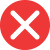12th

Correct spelling, explanation: the correct form is 12th, because nd is the abbreviation for second, therefore the numeral ending with nd must be pronounced with second at the end (for example 22nd; twenty-second). This is the reason why writing 12nd is incorrect and 12th is the correct form.

Definition of 12th:
numeral, the ordinal number between 11th and 13th
Jamie was 12th out of 100 in the cooking contest.
This is my 12th time in Spain.12nd

Incorrect spelling, explanation: this form is wrong because the correct pronunciation is twelfth, thus there is th at the end. This means that the correct writing of this numeral is 12th as in full-written form. Suffix nd is added only to these ordinal numbers which end with second, for example, 32nd, 102nd, 3452nd.12st

Incorrect spelling12rd

Incorrect spelling